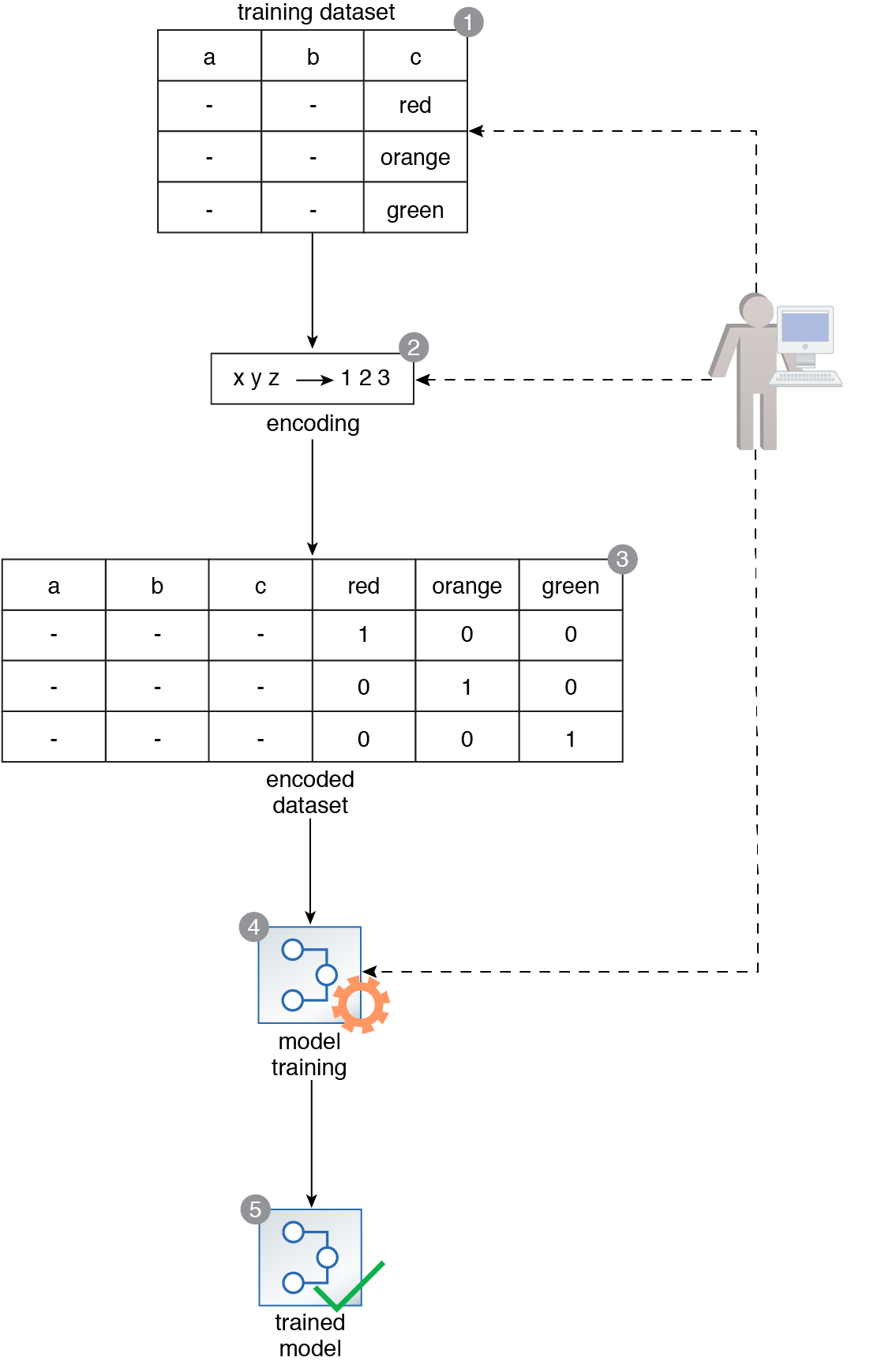# Feature Encoding

Artificial Intelligence (AI) Patterns, Neurons and Neural Networks > Data Wrangling Patterns > Feature Encoding

## Feature Encoding

How can categorical features be used for neural network development?

### Problem

Neural networks only support numerical features, which makes it impossible to use a dataset comprised of categorical features to develop a neural network.

### Solution

All categorical features in the dataset are converted to a numeric representation using a mathematical function.

### Application

The categorical features are encoded to numerical features using a specific encoding scheme, such as a label encoder and one hot encoder.A data scientist prepares a training dataset comprised of two numerical and one categorical feature to be used to train a neural network (1). The dataset’s categorical feature, c, is encoded using a label encoder (2) and then a one hot encoder (3). The encoded dataset now contains only numerical data where the categories become the features, such that the presence of each categorical value for a certain row is represented by a 1 while 0 represents its absence (3). The resulting dataset is then used to train the neural network without any issues (4, 5).